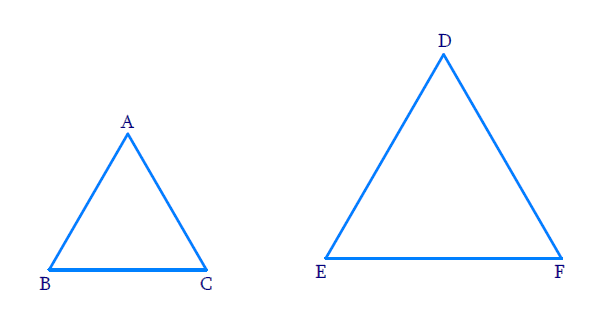# Ex.6.4 Q1 Triangles Solution - NCERT Maths Class 10

Go back to  'Ex.6.4'

## Question

Let $$\Delta ABC \sim \Delta DEF$$ and their areas be, respectively, $${64\,\rm{c}}{{\rm{m}}^{\rm{2}}}$$ and $${121\,\rm{c}}{{\rm{m}}^{\rm{2}}}$$. If $$E F=15.4\, \rm{cm}$$ find $$BC.$$

DiagramVideo Solution
Triangles
Ex 6.4 | Question 1

## Text Solution

Reasoning:

As we know that the ratio of the areas of two similar triangles is equal to the square of the ratio of their corresponding sides.

Steps:

$$\Delta ABC \sim \Delta DEF$$

\begin{aligned} \frac{\text { Area of } \Delta A B C}{\text { Area of } \Delta D E F} &=\frac{(B C)^{2}}{(E F)^{2}} \\ \\ \frac{64\, \rm{c m^{2}}}{121\, \rm{c m^{2}}} &=\frac{(B C)^{2}}{(15.4)^{2}} \\(B C)^{2} &=\frac{(15.4)^{2} \times 64}{121} \\ B C &=\frac{15.4 \times 8}{11} \\ B C &=11.2\, \mathrm{cm} \end{aligned}

Learn from the best math teachers and top your exams

• Live one on one classroom and doubt clearing
• Practice worksheets in and after class for conceptual clarity
• Personalized curriculum to keep up with school